Shapes
Random
Perimeter
Area
Perimeter & Area
100

If you were measuring the length of a finger with a ruler, what unit of measurement could you use??

HINT: You wouldn't use miles, feet, or yards....

You would use inches!

For example, Aubree's hand might be five and a half (5 1/2) inches!

100

What (five-sided) polygon is this?It is a pentagon!!

100

What is the perimeter? Is it the "outside" or the "inside?"

Also, do we add or multiply to find the perimeter??

The perimeter is the "outside."

And, we add to find the perimeter!

100

What is the area? Is it the "outside" or the "inside?"

Also, do we add or multiply to find the area??

The area is the "inside."

And, we multiply to find the area!

100

What month is Christmas in?

December!!

200

Draw two quadrilaterals. Explain how they are alike and different.

:)

200

True or False: Mr. Thorelli eats candy at his desk sometimes.

FALSE! He never eats any candy!

200

What is the perimeter of this shape?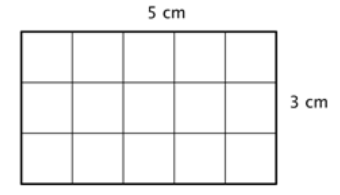You would do 5 + 5 + 3 + 3.

This equals 16cm! Try not to forget the labels even though Mr. T does sometimes.

Remember, showing your work is very important! If you just give me an answer, you will lose lots of points on the test.

200

What is the area of the following shape?Well, you would need to multiply 3 times 5...

So, 3 x 5 = 15 square cm

200

What is the area of this square?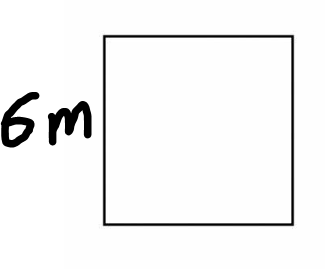The area would be 6 x 6 = 36 sq m.

300

Which team is never going to win another Super Bowl? (Because no one likes them.)

The Patriots (or the Packers.) Both teams are never going to win again!!!!

300

Fill in this line plot using the tally chart. Use Xs to show data on the line plot.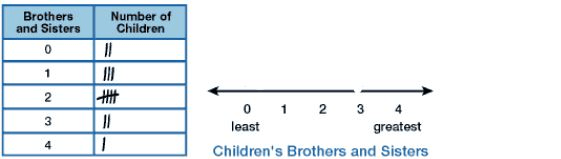300

What is the perimeter of the following figure?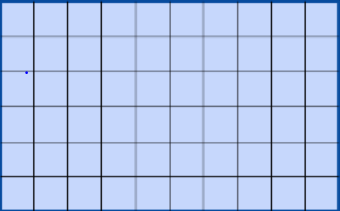Well, if you count by ones, you will see that there are six (6) rows and that there are ten (10) squares in each row.

So, 6 + 6 + 10 + 10= 32 units!

300

What is the area of the following figure?Well, we have six rows of ten.

So, we would do 6 x 10 which equals 60 square units!!

300

What is the area of the following figure?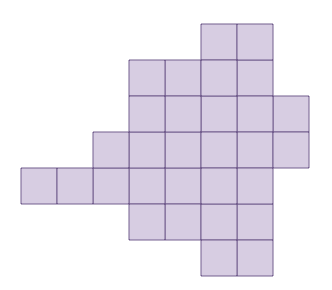You would count by ones! The area is 30 square units.

400

How are these following shapes alike?

How are they different?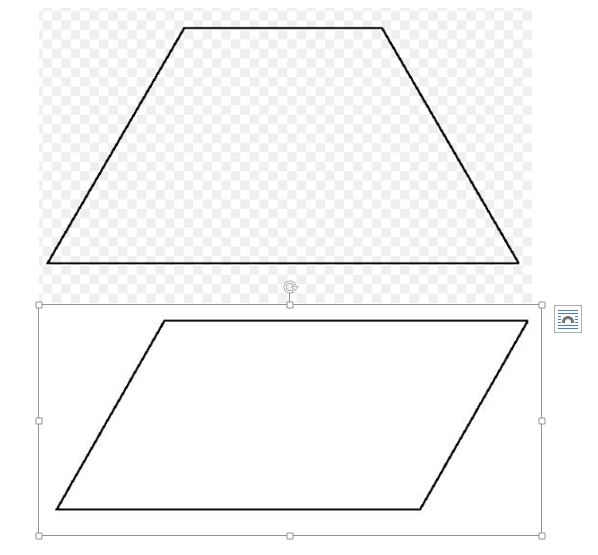They are both polygons, and they are both quadrilaterals.

The top shape is a trapezoid and has ONE set of parallel lines. The bottom shape is a parallelogram and has TWO sets of parallel lines.

400

What makes this shape a rectangle? (No, not because it has four sides or is a quadrilateral. There are many shapes with four sides that are not rectangles.)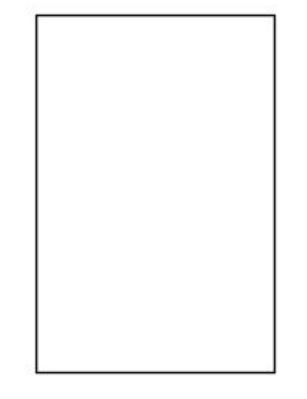What makes it a rectangle is that it has four right angles!

400

What is the perimeter of the following shape? HINT: It is a square.Well, since squares have four sides that are all the same length, that means that each side is 7 units.

So, 7 + 7 + 7 + 7 = 28 units!

400

What is the best mobile game?

Pokemon Go!!

400

What is the perimeter of the following figure?The perimeter would go as follows:

4 + 3 + 3 + 2 + 2 + 6 = 20 units!

500

Draw another shape that "Fits the Rule."

What do you think the rule is?The rule is: "It must be a rectangle."

All three shapes are quadrilaterals and parallelograms, but the two shapes that fit the rule are both rectangles (they have four right angles.)

500

Name this shape with as many names as possible. You need AT LEAST FOUR names. If you can come up with all SIX, you will get 1,000 points (instead of 500.)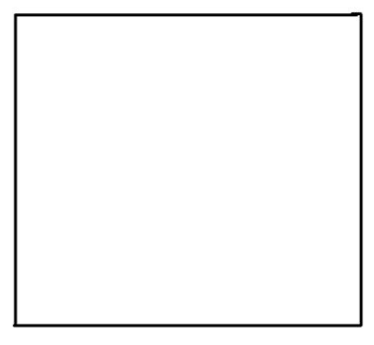1.) Polygon

3.) Parallelogram

4.) Rectangle

5.) Rhombus

6.) Square

500

What is the best name for a baby girl?

Olive!

500

What is the area of the figure below? You will get 1,000 points if you get this correct. HINT: Break it up into smaller rectangles!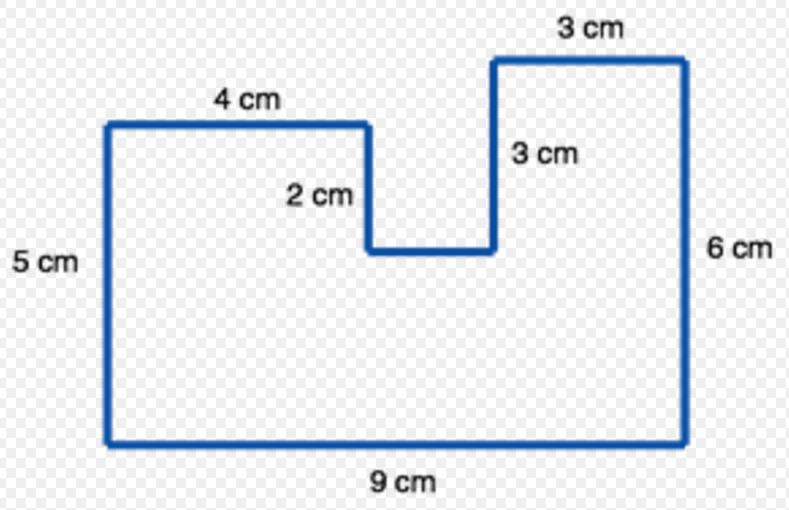Well, if you break it up into three rectangles, they would look like this: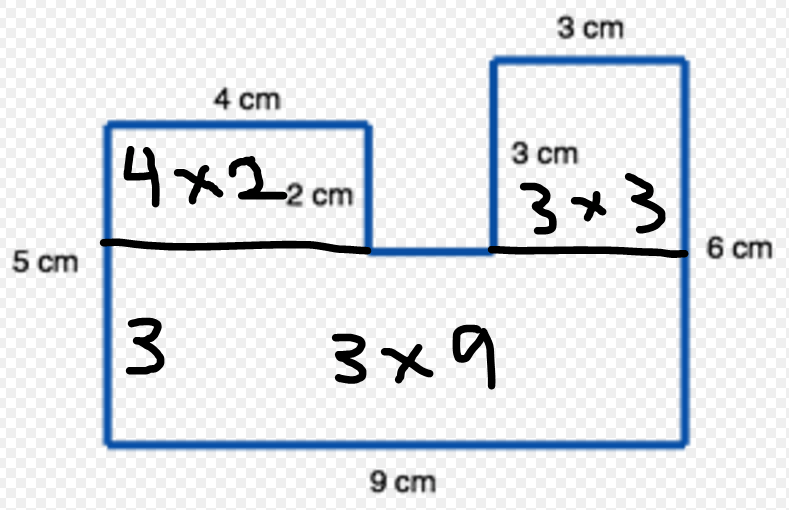So, you would have:

4 x 2 = 8, 3 x 3 = 9, and 3 x 9 = 27

8 + 9 + 27=44 sq cm!

500

What is the perimeter AND the area for the following parallelogram?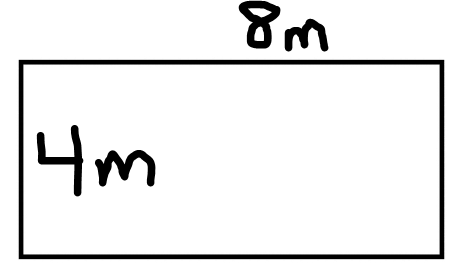Well, the perimeter would be 8 + 8 + 4 + 4 = 24 m.

The area would be 4 x  8 = 32 sq m.

Click to zoom